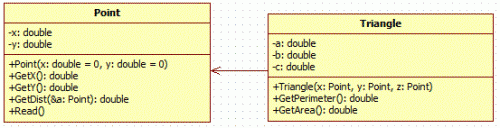Competitions

Perimeter and area of triangle

The real numbers x1, y1, x2, y2, x3, y3 - the coordinates of triangle vertices are given. Find the perimeter and the area of a triangle.Input

In one line the coordinates of triangle vertices are given: 6 numbers x1, y1, x2, y2, x3, y3 that do not exceed 100 by absolute value.

Output

Print in one line the perimeter and the area of a triangle. Print the answer with 4 decimal digits.

Time limit 1 second
Memory limit 128 MiB
Input example #1
3 2 7 6.5 10 1
Output example #1
19.3568 17.7500
Source SFE-2010 Variant 25